Courses
Courses for Kids
Free study material
Offline Centres
More

# Representing Data on Bar GraphLast updated date: 25th Nov 2023
Total views: 113.1k
Views today: 3.13k## Representing Data on Bar Graph: An Introduction

A bar graph is a chart or a graphical depiction of information, amounts, or numbers using bars or strips. To make a comparison of numbers, frequencies, or other measurements of different categories of data, bar graphs are used. The bars offer a visual representation for comparing quantities in different categories. Bar graphs contain the x and y axes, also referred to as the horizontal and vertical axes, as well as the title, labels, and bars. Each bar's height can be used to display information such as the number of items in each category or the frequency of an event. Let's describe what a bar graph is, how kids are taught to recognise them, how to make a bar graph on graph paper, and how to make one with groups of discrete data.

## Types of Bar Graph

There are mainly two types of bar graphs: horizontal graph and vertical graph.

1. Vertical Bar Graphs: Vertical bar graphs are those in which the given data are displayed vertically in a graph or chart with the aid of rectangular bars that display the measure of data. The y-axis displays the value of the height of the rectangular bars, which indicates the quantity of the variables written on the x-axis, and the rectangular bars are drawn vertically on the x-axis.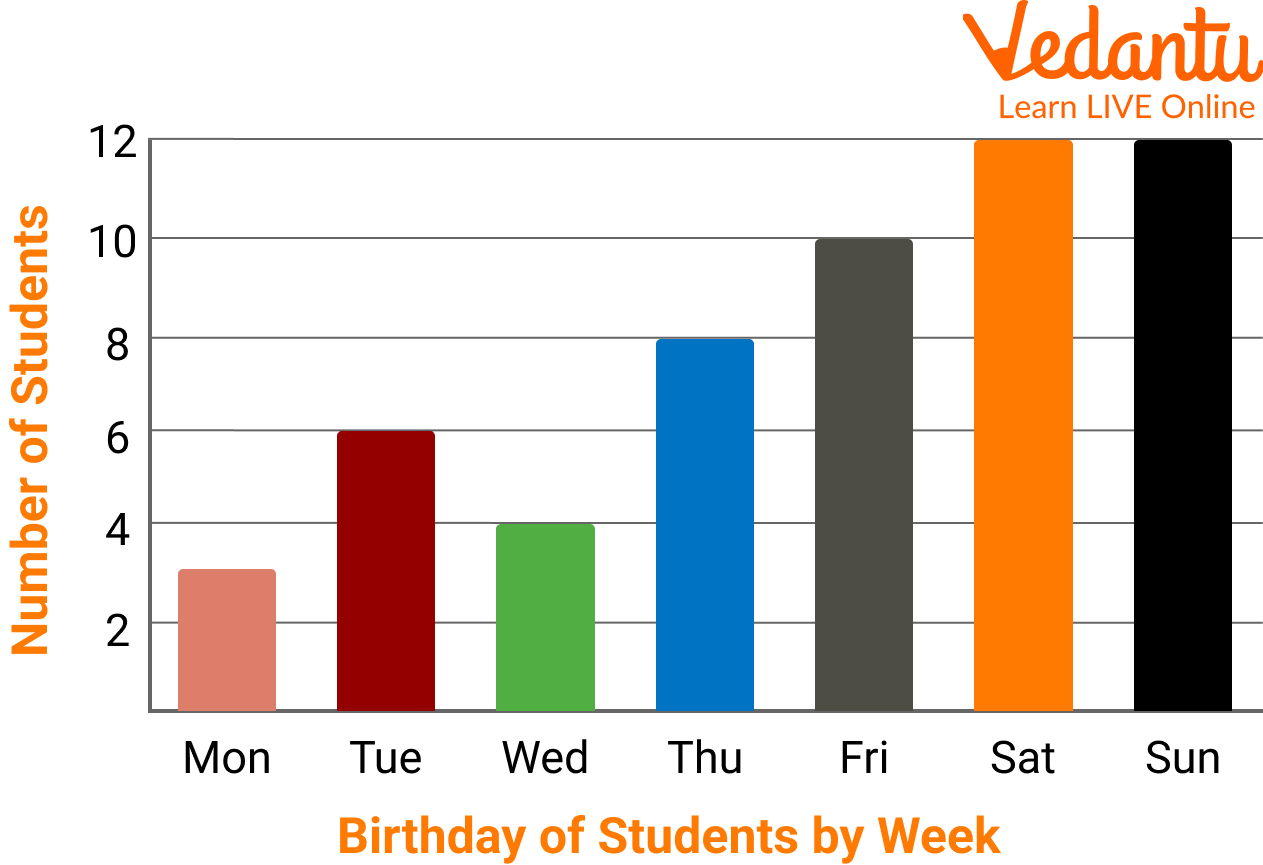Vertical Bar Graphs

1. Horizontal Bar Graphs: These graphs are referred to as horizontal bar graphs when the given data is displayed horizontally by employing rectangular bars that display the data's measure. In this type, the categories or variables of the data must be put down before the rectangular bars are drawn horizontally on the y-axis and the length of the bars is displayed on the x-axis as the values of the various variables present in the data.Horizontal Bar Graphs

## Bar Graph of Population

Create a bar graph using the information below.

India's population in each of the subsequent census years:

 Year 1941 1951 1961 1971 1981 1991 2001 Population (in millions) 320 360 440 550 680 850 1000

We use the years on the X-axis and the population on the Y-axis to build the graph. We plot the graph in rectangles each year by assuming that both axes have a scale, which implies that we use 10 years as one unit, which will be the width of the rectangle, and the population as the length of the rectangle.

Let's now establish a table with the coordinates of the points that will be shown on the graph in one column, as shown below.

 Year Population(in millions) Co-ordinates 1941 320 (1941, 320) 1951 360 (1951, 360) 1961 440 (1961, 440) 1971 550 (1971, 550) 1981 680 (1981, 680) 1991 850 (1991, 850) 2001 1000 (2001, 1000)

Let's use the scale now.

10 years are equal to 1 unit on the X-axis.

One unit equals 100 million on the Y-axis.

Let's plot the given data's bar graph using this scale.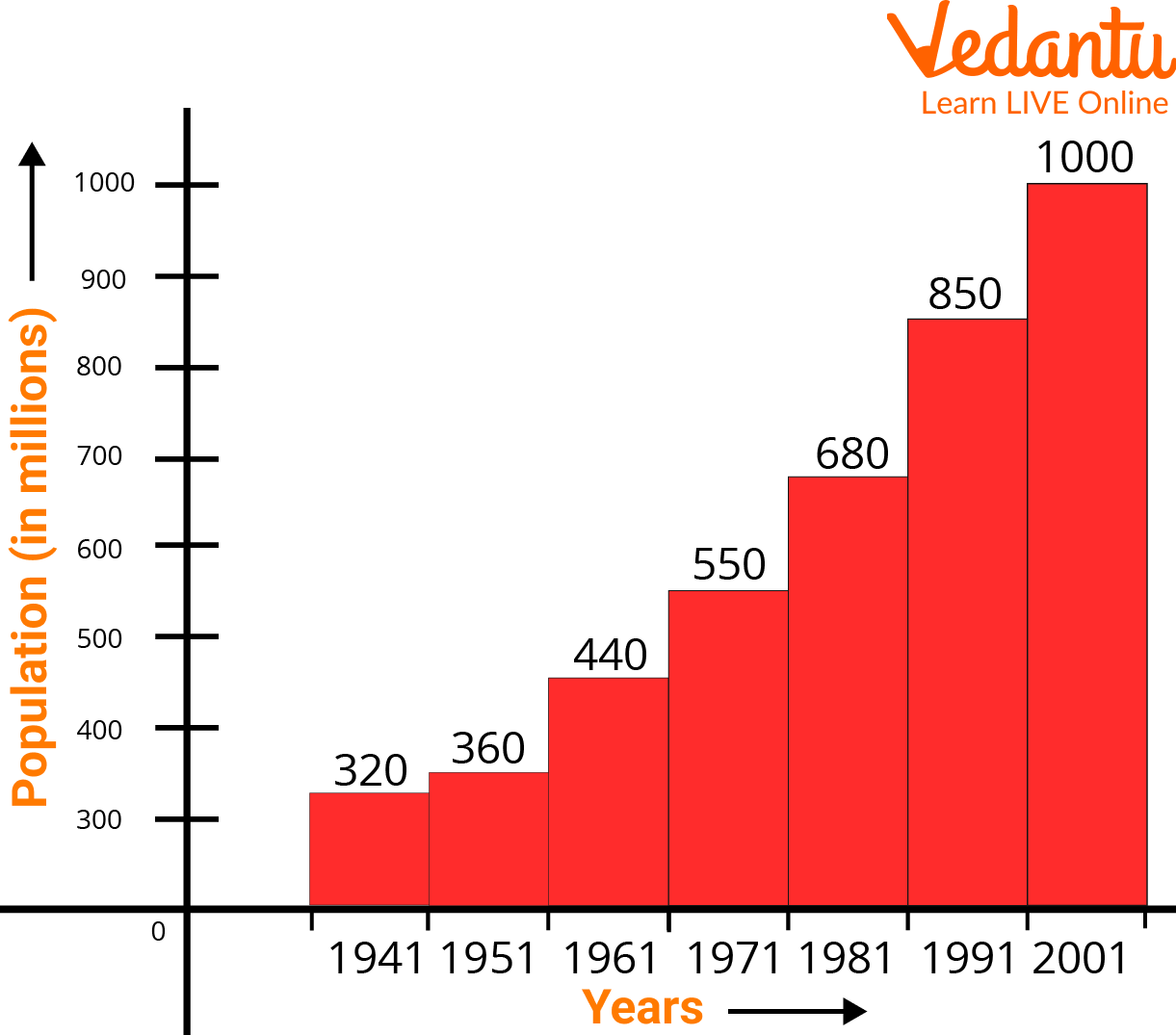Bar Graph of Population

As a result, a bar graph showing the provided data is created.

## How to Draw a Bar Graph on a Graph Paper?

With the aid of an example, let's learn how to draw a bar graph. Liza visited the market to purchase a variety of fruits, including 5 apples, 3 mangoes, 2 watermelons, 3 strawberries, and 6 oranges. She wants to create a bar graph to visualize the data so she can see what kinds of fruits she purchases the most frequently.

Let's use the next few stages to create a bar graph of the fruit that is most frequently purchased.

• Step 1: Take a piece of graph paper, and label the bars with a caption like "Most Bought Fruit."

• Step 2: On a plane, draw the horizontal (x-axis) and vertical (y-axis) axes.

• Step 3: Next, give the horizontal axis, which is an independent category, the name "Types of Fruits," and the vertical axis, which is a dependent category, the name "Number of Fruits."

• Step 4: Write the names of the fruits on the horizontal axis, such as apples, mangoes, watermelon, strawberries, and oranges, and leave an equal gap or spacing between each fruit.

• Step 5: Describe the graph's scale, which demonstrates how numbers are used in the data. It is a system of markers spaced at specific intervals that aids in object measurement. The scale of a graph, for instance, could be expressed as 1 unit = 1 fruit.

• Step 6: Start constructing rectangular bars for each fruit, leaving equal spaces between them, and assigning heights to the corresponding numbers.

• Step 7: Now that the bar graph is complete, determine which fruit is the most popular by measuring the height of the rectangle bars for each fruit.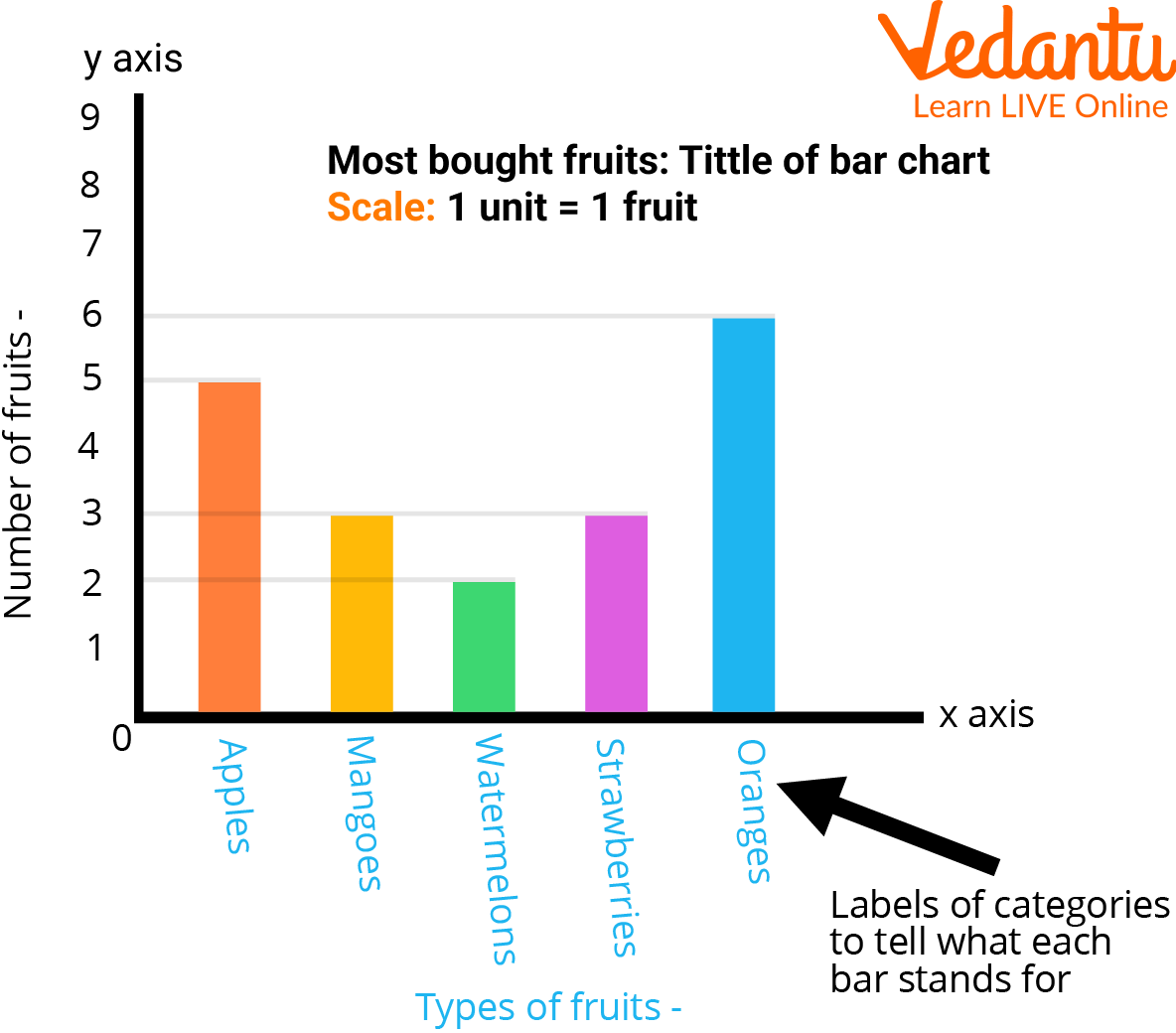Bar Graph on Graph Paper

We may conclude from the graph above that Liza purchases oranges more frequently than any other fruit.

## Green Bar Graph Paper

A piece of writing paper with thin lines printed on it to create a regular grid is known as graph paper, coordinate paper, grid paper, or squared paper. When producing graphs of functions or experimental data and sketching curves, the lines are frequently used as a guide. The green graph is one of the most often used graph papers with color-coded labels that you'll find. Graph paper is a generic term for a sheet of paper with grids or fine lines on it. These tiny lines are what give the paper's grid its ultimate shape as a graph. We use green graph paper to make bar graphs.Green Graph Paper

### Solved Examples

Example 1: We surveyed 100 youngsters to find out about their preferred method of transportation to school. The table below lists the findings of this survey. Draw a bar graph to illustrate this data.

 Mode of Transportation Frequency Walking 29 Cycling 15 Car 35 Bus 18 Train 3

Ans: We must first determine from the data in the table what our graph's requirements will be before we can create the bar graph:The y-axis scale must extend at least as high as 35 because there are 5 categories and there must be space for 5 bars. The highest frequency is 35.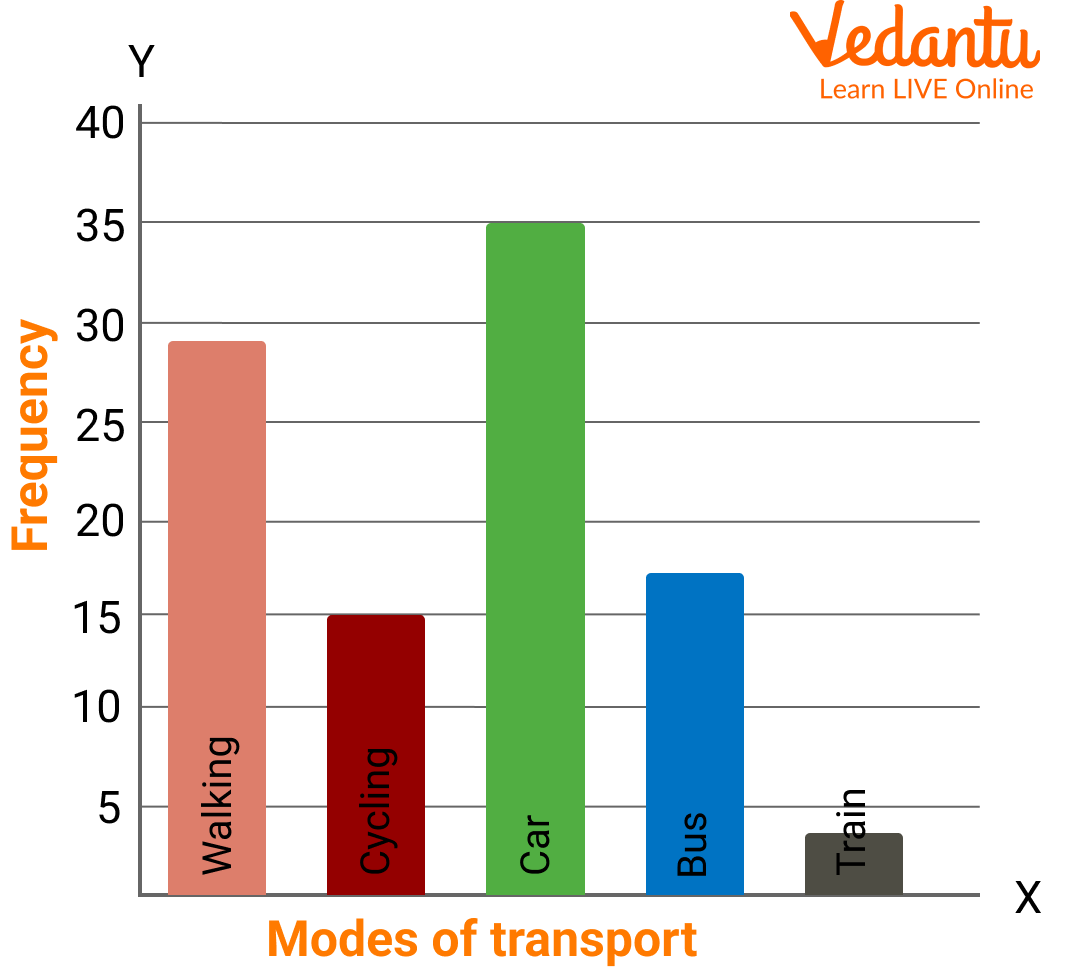Bar Graph

Example 2: The data of a student's class test is given below. Draw a bar graph to illustrate this data.

 Grade A B C D Students 4 12 10 2

Ans: The bar graph of the given data is given below:Bar Graph

### Practice Problems

1. A survey of 5 bike companies was conducted to determine "Which company sold the maximum bikes?"

 Bike Company Hero Honda BMW Yamaha TVS Number of bikes sold 35 30 10 25 40

Draw a horizontal bar graph for the given data.

Ans: Bar graph for the given data is: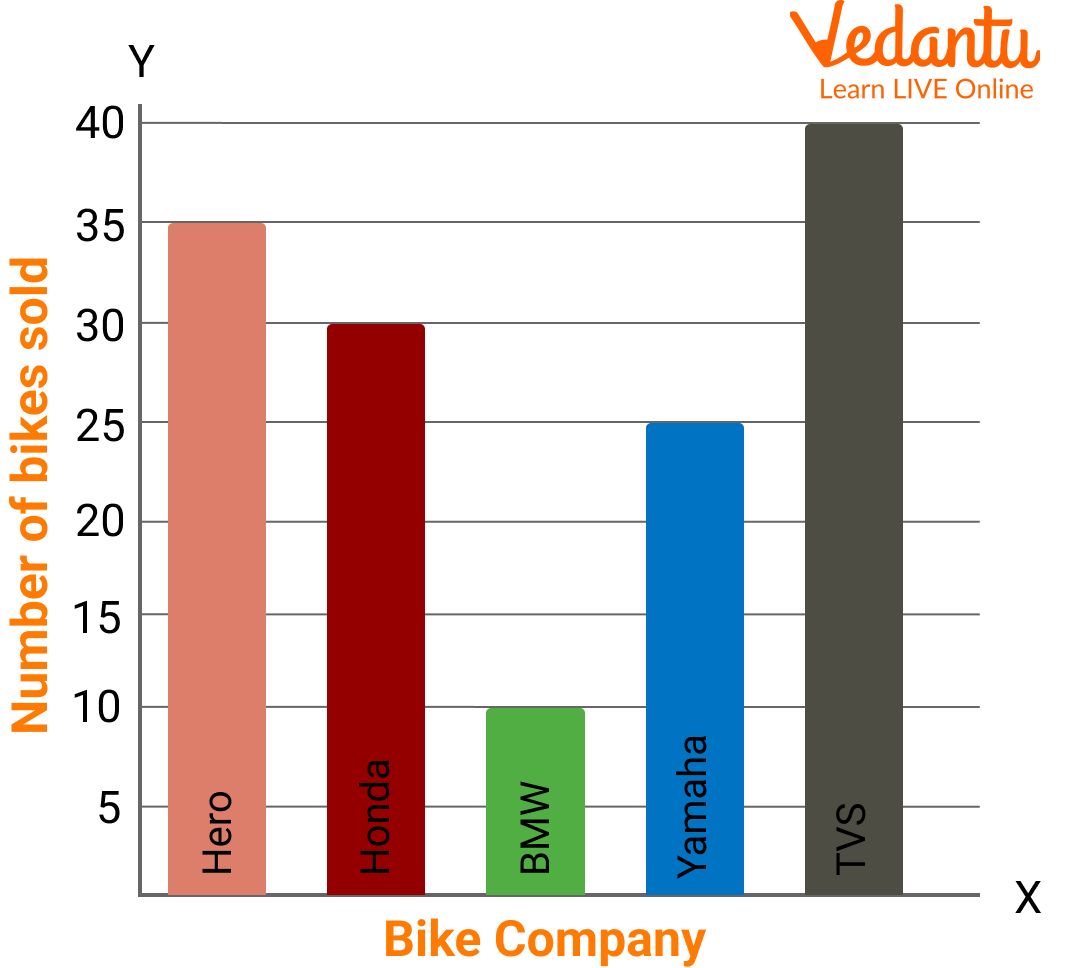Bar Graph

TVS sold the maximum bike.

## Summary

In this article we learned about a bar chart, often known as a bar graph, which is a diagram that displays categorical data as rectangular bars with heights or lengths proportional to the values they stand for. Bar graphs and charts give categorical data a visual representation. Categorical data is the categorization of data into distinct groups, such as age range, shoe size, and animal species. Typically, these classifications are qualitative.

## FAQs on Representing Data on Bar Graph

1. What are the characteristics of a bar graph?

Among the graphs, it is the most straightforward graphical representation of the data that uses columns or rectangles with equal widths to depict the data. The following list includes some characteristics that distinguish a bar graph apart from other types of graphs:

1. The width and spacing between each rectangular bar should be the same.

2. Either horizontally or vertically can be used to draw the rectangular bars.

3. The rectangular bars' height is equal to the height of the data they depict.

4. There must be a common base for the rectangular bars.

2. What distinguishes a line graph from a bar graph?

A line graph is a type of graph in which a sequence of data points are connected by a line, whereas a bar graph is a particular technique of showing data using rectangular bars, with the height of each bar being proportional to the value it displays in data. Bar graphs show correlations between the data rapidly, but line graphs place an excessive number of lines across the graph and can occasionally be difficult to read.

3. What is the difference between a bar graph and a histogram?

The bars in a bar graph are not next to one another, which is the reason histograms and bar graphs differ from one another. On the other hand, two consecutive bars on a histogram are close together. In a bar graph, the spaces between the two consecutive bars must be equal, however in a histogram, the bars must be joined together and the X-axis must only display continuous data expressed as numbers.